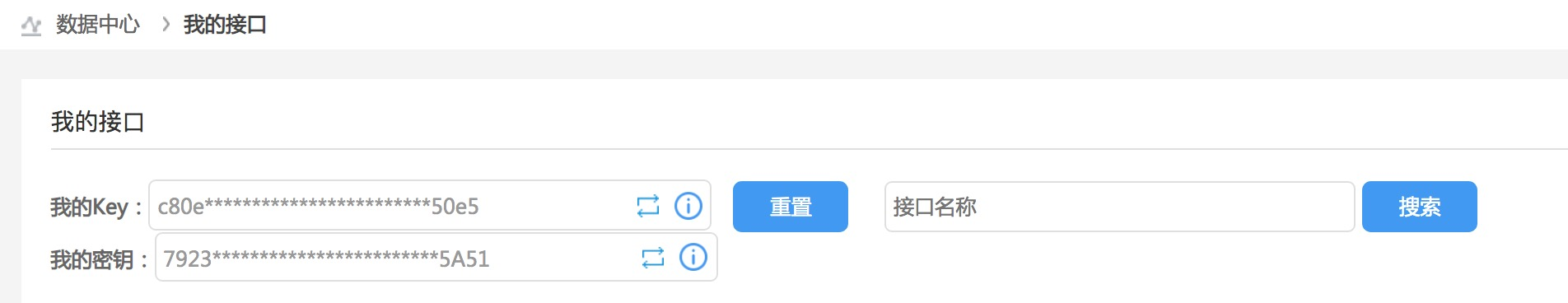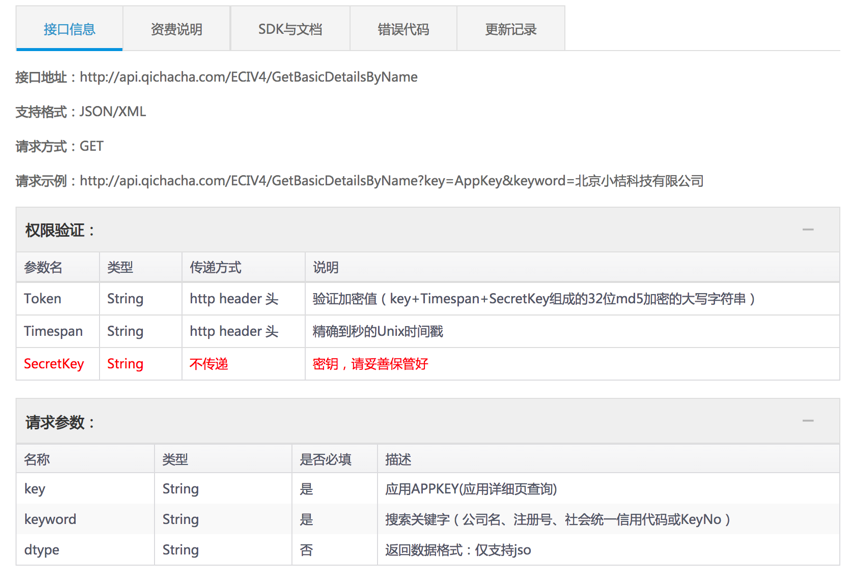# 企查查api接口批量操作实战

1、了解需求，申请接口。2、了解请求参数设置以及返回数据的格式1）这里请求参数中key就是我的key

keyword就是查询的关键字（企业名称、注册号、社会统一信用代码）

Timespan就是精确到秒的时间戳。

Token就是将我的key、精确到秒的时间戳、我的密钥三个东西拼接，然后使用md5加密算法进行加密，加完密之后再所有字符串全部转化为大写。

2）返回的数据一般分两种，有些接口只返回json格式的数据。我们直接解析提取相应格式获取数据就可以。

3、代码实现

import requests
import json
import time
from hashlib import md5

－－－－－－－－－－－－－－－
#获取时间戳
def get_time_tup():
"""
:return: 13位精确到秒的时间戳
"""
time_tup=str(int(time.time()))
return time_tup
－－－－－－－－－－－－－－－－－－－－
#md5加密
def set_md5(s):
"""
:param s: 拼接的字符串
:return: md5加密再转化为大写的字符串
"""
new_md5 = md5()
new_md5.update(s.encode(encoding='utf-8'))
s_md5 = new_md5.hexdigest().upper()
return  s_md5
－－－－－－－－－－－－－－－－－－－－－－
#设置请求头
"""
:param key: 我的key
:param screat_key: 我的密钥
:return: 请求头
"""
token=key+get_time_tup()+screat_key


－－－－－－－－－－－－－－－

#批量申请数据

def get_data(codes,name,key,screat_key):
"""
:param codes: 关键字的可迭代对象
:param name: 存储文档的署名
:param key:  我的key
:param screat_key: 我的密钥
:return:  无
"""
with open(f"data_{name}.csv", "w") as f,open(f"error_data_{name}.csv","w") as f2:
count=0
count_true = 0
count_false = 0
for code in codes:
try:
count+=1
url = f"http://api.qichacha.com/ECIV4/GetBasicDetailsByName?key={key}&keyword={code}"
t=s.text
print(m)
if m["Status"]=="200":
x=m["Result"]
f.write(str(x["KeyNo"])+'~'+str(x["Name"])+'~'
+str(x["No"]) + '~'+str(x["BelongOrg"]) + '~'
+str(x["OperName"]) + '~'+str(x["StartDate"]) + '~'
+str(x["EndDate"]) + '~' +str(x["Status"]) + '~'
+str(x["Province"])+ '~' +str(x["UpdatedDate"]) + '~'
+str(x["CreditCode"]) + '~'+str(x["RegistCapi"]) + '~'
+str(x["Scope"]) + '~' +str(x["TermStart"]) + '~'
+str(x["TeamEnd"])+ '~'+str(x["CheckDate"]) + '~'
+str(x["OrgNo"]) + '~'+str(x["IsOnStock"]) + '~'
+str(x["StockNumber"]) + '~'+str(x["StockType"])
+"\n")
count_true += 1
else:
f2.write(code)
f2.write("\n")
count_false+=1
except :
f2.write(code)
f2.write("\n")
count_false+=1
print("匹配到的数据",count_true)
print("未匹配到的数据",count_false)
print("数据总数",count)
if __name__ == '__main__':
＃此处需要自己调整
codes=data["企业名称"]
key=我的key
screat_key=  我的密钥
get_data(codes, "example", key, screat_key)

10-19
06-1359806-307866
02-2228
07-21345
11-281万+
07-17262
01-023316
04-051642